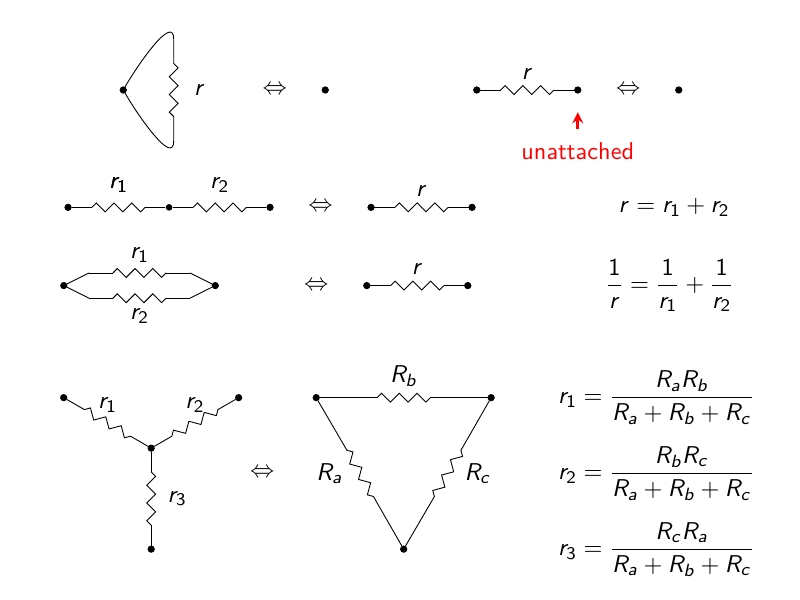#### June 28-29, 2015

Here are the slides for two related talks: one on control theory and one on electrical circuits, both from a category-theoretic perspective.

### Categories in ControlControl theory is the branch of engineering that studies dynamical systems with inputs and outputs, and seeks to stabilize these using feedback. Control theory uses 'signal-flow diagrams' to describe processes where real-valued functions of time are added, multiplied by scalars, differentiated and integrated, duplicated and deleted. In fact, these are string diagrams for the symmetric monoidal category of finite-dimensional vector spaces, but where the monoidal structure is direct sum rather than the usual tensor product. Jason Erbele has given a presentation for this symmetric monoidal category, which amounts to saying that it is the PROP for bicommutative bimonoids with some extra structure.

A broader class of signal-flow diagrams also includes 'caps' and 'cups' to model feedback. This amounts to working with a larger symmetric monoidal category where objects are still finite-dimensional vector spaces but the morphisms are linear relations. Erbele also found a presentation for this larger symmetric monoidal category. It is the PROP for a remarkable thing: roughly speaking, an object with two special commutative dagger-Frobenius structures, such that the multiplication and unit of either one and the comultiplication and counit of the other fit together to form a bimonoid.

You can see the slides here. Also try:

### Circuits, Categories and Rewrite RulesWe describe a category where a morphism is an electrical circuit made of resistors, inductors and capacitors, with marked input and output terminals. In this category we compose morphisms by attaching the outputs of one circuit to the inputs of another. There is a functor called the 'black box functor' that takes a circuit, forgets its internal structure, and remembers only its external behavior. Two circuits have the same external behavior if and only if they impose same relation between currents and potentials at their terminals. This is a linear relation, so the black box functor goes from the category of circuits to the category of finite-dimensional vector spaces and linear relations. Constructing this functor makes use of Brendan Fong's theory of 'decorated cospans' — and the question of whether two 'planar' circuits map to the same relation has an interesting answer in terms of rewrite rules.

You can see the slides here. Also try: